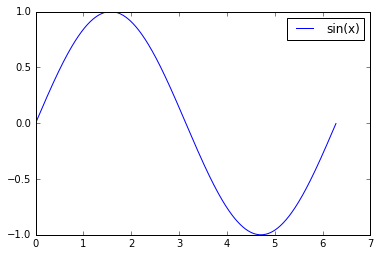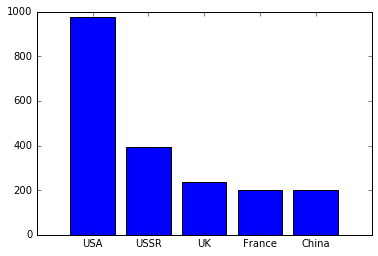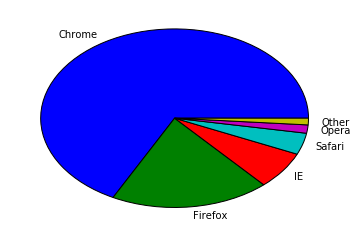# Introduction to Matplotlib

Standard

In the previous entry, we introduced the libraries available for graphing in Python. In this entry, we will have a very basic look on the first proposed library, Matplotlib. We will create three types of graphics: a line plot, a bar chart and a pie chart.

We start by creating the data for the line chart. In this case, we will draw a sinus function using the Numpy library. Line plots are usually fit for data where one (or more) dependent variables are represented as a function of an independent variable. A typical example are variables that change over time or a spatial dimension. Usually, the independent variable is a continuous variable.

In :
%matplotlib inline
import numpy as np
import matplotlib.pyplot as plt

x = np.arange(0,2*np.pi,0.01)
y = np.sin(x)


We have created a range from 0 to 2$\pi$, with a step of 0.01 by calling np.arange(), and then we just call the np.sin() with the resulting array to calculate the sinus. We then have two variables x and y that hold the axis and the values of the function. We now plot them with Matplotlib.

In :
plt.figure()
plt.plot(x,y)
plt.legend(['sin(x)'])
plt.show()We first open a new figure (pretty much like in Matlab), proceed to create the line plot, and add a legend for the only dependent variable. Finally, we explicitly tell Matplotlib to draw the plot on screen (in this case, since we are using Jupyter and we have used the %matplotlib inline magic, the plot will be drawn in the notebook).

Next, we will draw a bar plot. Bar plots are mostly used to compare the value of a certain variable over a finite (and ideally small) number of classes. For instance, we can show the number of gold medals earned by the top 5 countries on all Olympic Games.

In :
top_5 = ['USA','USSR','UK','France','China']
medals = [976, 395, 236, 202, 201]


This is the format that our data must take in order to draw the plot. We will just consider that we have the data already formatted, although normally it will not be given like this (it will come in large data frames or CSV files for example, but more on that in later entries); and it is not even recommended to use this format (two separate lists). We will now draw the barplot. This code may not be very beautiful…

In :
plt.figure()
plt.bar(range(5),medals, align='center')
ax = plt.gca()
ax.set_xticks(range(5))
ax.set_xticklabels(top_5)
plt.show()In the first line, we create a new figure. Next, we draw the barplot. We must give some x axis values, so we call range(5), that creates a list of integers from 0 to 4; and it is used as the first argument of plt.bar(). The second argument is our data, and the third argument tells plt.bar() to center each bar over the tick (the numbers from 0 to 4). Next, we must change the ticks in order to reflect the country names. We must first get a handle on the axes of the plot with ax = plt.gca(). With the axis, we must first set the ticks again with the call to ax.set_xticks(range(5)), otherwise the country names will be misaligned. Finally, we change the numeral ticks to the country names and paint the plot.
The code is not simple to understand (and trust me, it is not simple to explain either). Working with Matplotlib there is a lot of documentation research and trial and error in order to obtain correct graphics, which makes it sometimes a frustrating experience.

Next, we are going to draw a pie chart. Pie charts are normally used to show how a total quantity is divided among several classes. For instance, the proportions of votes in an election, or the usage of web browsers among users. We will use the data for November 2015.

In :
browsers = ['Chrome', 'Firefox', 'IE', 'Safari', 'Opera']
users = [67.4, 19.2, 6.8, 3.9, 1.5]


This data does not sum 100%, so there is incomplete data. We will assume in this case that the remaining percentage is Other.

In :
browsers.append('Other')
users.append(100-sum(users))


We proceed now to paint the graphic.

In :
plt.figure()
plt.pie(users,labels=browsers)
plt.show()Again, we create the figure, we call the plt.pie() method, passing the data as the first argument and the labels for each value as the second parameter. We then order Matplotlib to paint the graphic.
The colors may not be too representative of the browsers, but we will leave that for the next entry, where we will decorate and improve these examples.

In this entry we have seen a basic example of three very common types of graphics. There are many others, but if would take a whole site to show all the available types. The results with the minimal code are very basic graphics that needs some work in order to improve the presentation.

This site uses Akismet to reduce spam. Learn how your comment data is processed.